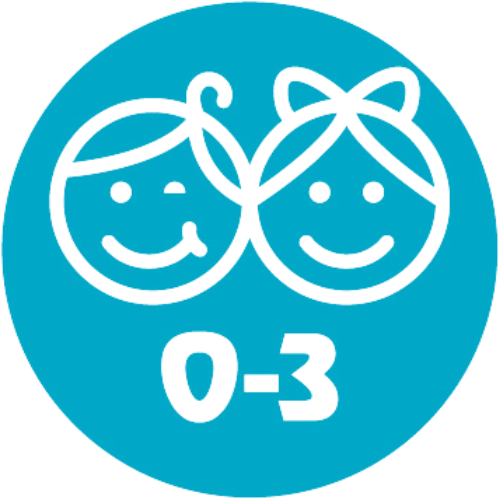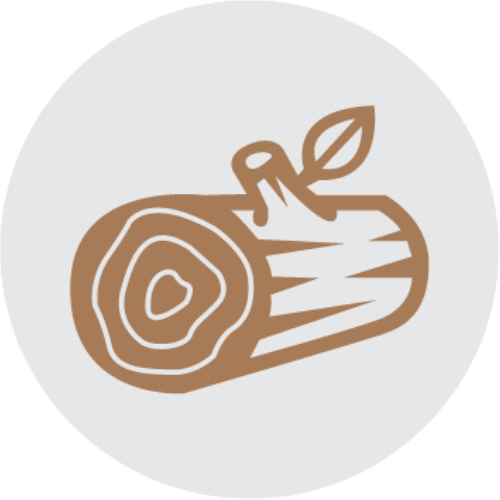# SumBlox Intro Set of 38

SKU: 331-011
\$166.99
Taxes and shipping calculated at checkout
In stock and ready for shipping#### Sustainable#### Ages 0-3#### Wood

For budding makers and mathematicians alike, SumBlox are an ingenious way to explore the foundations of mathematics in 3 dimensions. Sumblox are a hands-on transition from concrete to semi-concrete mathematical thinking.

Carefully manufactured from natural wood they're an ideal addition to any learning environment from pre-school to middle-school. Includes 43 hardwood blocks and activity card. Ages 2+.

##### Contents
• Fourteen  -1 blocks
• Six - 2 block
• Four - 3 block
• Three - 4 block
• Two each of  -5, 6, 7, 8, 9
• One  -10
• Activity Cards

SumBlox are math building blocks whose individual size is proportional to the number value. Put a "1" block on top of another "1" block and it is the same height as the "2" block. Stack three "3's" beside a "9" and you'll notice they come up to the same level.

Through hands on play, Sumblox engages and excites children to visually represent math in a unique and fun way that promotes collaboration and cooperation. Stack proportional number blocks and they are literally equal in size and value! SumBlox help with developing understanding of addition, fractions, composing and decomposing, multiplication and so much more!

SumBlox Building Blocks teach a wide range of mathematics through guided, hands-on discovery in our SumBlox Learning Activities. Currently available are series that teach addition and subtraction, multiplication, and fractions. Each series empowers the educator to gradually move students to a deeper understanding of the properties of math through step-by-step investigations, engaging discussions and fun challenges.

With Sumblox, students solidify their understanding of addition by focusing on its properties and manipulating the addend pairs of ten. Students connect addition to its inverse property, subtraction, and develop a strategy to find the difference between two whole numbers. These new skills are learned and assessed through fun activities like building the Tower of Ten.

Multiplication
Students use SumBlox to investigate factors and multiples. Multiplication comes to life as they physically see the relationship between multiplication and repeated addition. As they dig deeper into multiplication, they discover the commutative property and build SumBlox Factor Walls to discover all of the factors of a number.

##### What mathematical concepts can SumBlox teach?

Students can learn a wealth of information through play and hands-on exploration. These topics include:

#### Number Sense

• Number recognition
• One-to-one correspondence
• Compare numbers
• Subitizing
• Counting
• Cardinality
• Number sequence
• Hierarchical Inclusion
• Understanding our base-ten system
• Place value
• Measuring Lengths
• Reason abstractly and quantitatively
• Understand Equivalence
• Directly compare two objects with a measurable attribute in common
• Algebraic Thinking
• Describe measurable attributes of objects, such as length or weight
• Classify objects into given categories

• Solve Addition and Subtraction word problems using objects
• Compose and decompose numbers
• Skip counting

#### Multiplication / Division

• Define multiplication; emphasis on multiples
• Find factors of whole numbers and use commutative property of multiplication
• Classify whole numbers (greater than one) as prime or composite
• Draw conclusions about the factors of a whole number
• Use associative property of multiplication
• Use distributive property of multiplication

• Define denominator of fractions
• Define numerator of fractions within halves, fourths, and eights
• Find equivalent fractions within thirds, sixths, ninths, and twelfths
• Compare two fractions using inequality symbols
• Compare and order three fractions using compound inequalities
• Find the value of a fraction of a whole number
• Find the fraction, or part, a given value is of a whole value
• Add two fractions with the same denominator to get a sum up to one whole and fully decompose a given fraction
• Add two fraction addends with the same denominator to get a sum greater than one whole
• Add multiple fraction addends to find sums greater than one whole and represent sums in improper form and mixed numbers
• Add two fractions addends with different denominators (having to scale all addends to find a common denominator)

### Recommended for You## The Louise Kool Difference

Proven expertise, quality goods, guaranteed service